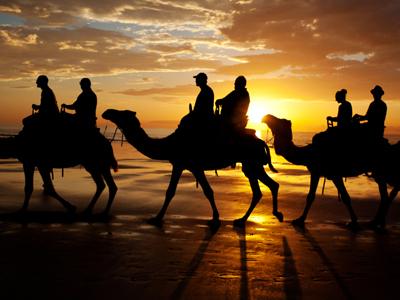Camels have big feet so they have a large area and the pressure is small. This means they don't sink into soft sand.

# Pressure 2

This Science quiz is called 'Pressure 2' and it has been written by teachers to help you if you are studying the subject at middle school. Playing educational quizzes is a fabulous way to learn if you are in the 6th, 7th or 8th grade - aged 11 to 14.

It costs only \$12.50 per month to play this quiz and over 3,500 others that help you with your school work. You can subscribe on the page at Join Us

Pressure is measured in Pascals. To calculate the area a force acts on to produce a pressure, you need to use the following equation: area = force/pressure.

See how well you do in our second Science quiz on the subject.......no pressure!

1.
A student weighs 500N, standing on two feet she exerts a pressure of 25,000Pa on the ground. What is the area of one foot?
50cm2
0.005m2
0.01m2
0.02m2
Area = 500/25,000 = 0.02 (two feet), one foot = 0.01m2
2.
Camels have big feet so they don't sink into soft sand because .......
they have a large area so the pressure is large
they have a large area so the pressure is small
they have a small area so the pressure is large
they have a small area so the pressure is small
3.
A box weighs 20N. It exerts a pressure of 2Pa on a table. What is the area of the box in contact with the table?
10cm2
0.1m2
10m2
40m2
Area = force/pressure = 20/2 = 10m2
4.
Doubling the force and doubling the pressure mean .......
that area doubles
that area gets larger
that area gets smaller
that area stays the same
5.
One box weighing 60N is put on top of one weighing 120N. The pressure they exert is 180Pa. What is the area touching the ground?
0.67m2
1m2
2m2
3m2
Area = (60 + 120)/180
6.
A 60,000N elephant can stand on one foot! The pressure it exerts is 240,000Pa. What is the area of its foot?
0.16m2
0.24m2
0.25m2
4m2
Area = 60,000/240,000
7.
A sharp knife cuts well because .......
it has a large area so pressure is large
it has a large area so pressure is small
it has a small area so pressure is large
it has a small area so pressure is small
8.
A skier weighs 600N and exerts a pressure of 2,500Pa on the snow. What is the area of the skis?
0.12m2
0.24m2
1.2m2
4.2m2
Area = 600/2,500 = 0.24m2
9.
A box weighs 100N. It exerts a pressure of 25Pa on a table. What is the area of the box in contact with the table?
4mm2
4cm2
4m2
4km2
Area = force/pressure = 100/25. Remember weight is a force
10.
A skier weighs 600N, lifts one ski up and exerts a pressure of 5,000Pa on the snow. What is the area of one of the skis?
0.12m2
0.24m2
0.48m2
1.2m2
Area = 600/5,000
Author:  Sue Davison